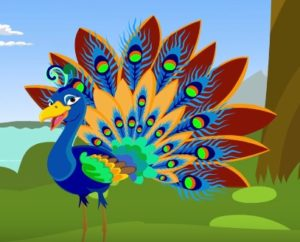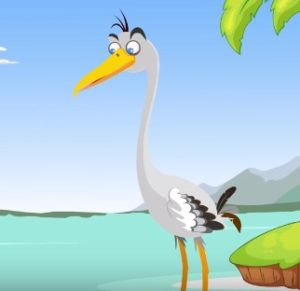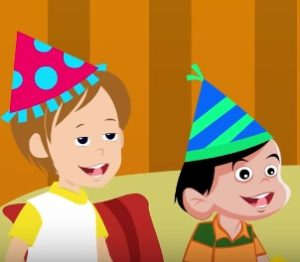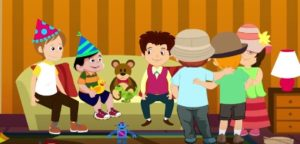Watch the video given below and then answer the questions

Questions

Nursery/LKG (Q# 1-3)

Q1 – Name the animal that you see in the image.Q2 – What is the animal that you see in the image?Q3 – What is the shape of the caps that the kids are wearing?LKG/UKG (Q# 4-5)

Q4 – How many kids do you see in the image?Q5 – What is a female peacock called?

UKG/1st (Q# 6-7)

Q6 – Find the odd one out and also state the reason: 4, 8, 12, 13, 16, 20

Q7 – Complete the equations:

• 9 ? 6 = 3
• 6 ? 7 = 13
• 18 – 9 = ?

Activity Time – Let’s explore the letter P

List down the names of at least 4 eatables whose name start with the letter “P”.

.

.

.

.

.

.

.

.

.

A1 – A Peacock.

A2 – A Crane.

A3 – The caps are conical in shape.

A4 – 6.

A5 – A Peahen.

A6 – 13 because it is the only odd number in the series.

A7 –  The equations are as follows:

• 9 – 6 = 3
• 6 + 7 = 13
• 18 – 9 = 9